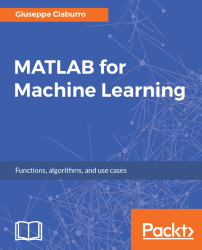•#### MATLAB for Machine Learning#### Overview of this book

MATLAB is the language of choice for many researchers and mathematics experts for machine learning. This book will help you build a foundation in machine learning using MATLAB for beginners. You’ll start by getting your system ready with t he MATLAB environment for machine learning and you’ll see how to easily interact with the Matlab workspace. We’ll then move on to data cleansing, mining and analyzing various data types in machine learning and you’ll see how to display data values on a plot. Next, you’ll get to know about the different types of regression techniques and how to apply them to your data using the MATLAB functions. You’ll understand the basic concepts of neural networks and perform data fitting, pattern recognition, and clustering analysis. Finally, you’ll explore feature selection and extraction techniques for dimensionality reduction for performance improvement. At the end of the book, you will learn to put it all together into real-world cases covering major machine learning algorithms and be comfortable in performing machine learning with MATLAB.
Title PageCreditsForewordwww.PacktPub.comCustomer FeedbackPrefaceFree Chapter
Getting Started with MATLAB Machine LearningImporting and Organizing Data in MATLABFrom Data to Knowledge DiscoveryFinding Relationships between Variables - Regression TechniquesPattern Recognition through Classification AlgorithmsIdentifying Groups of Data Using Clustering MethodsSimulation of Human Thinking - Artificial Neural NetworksImproving the Performance of the Machine Learning Model - Dimensionality ReductionMachine Learning in Practice## Importing data into MATLAB

In data analysis, a fundamental role is assumed by the exchange of such data between the analysis environment and external devices. We start from data collection and importing in MATLAB for whatever analysis we are going to do--always. As well, we will finish our activities by exporting the results. There are several ways to import and export data in the MATLAB environment depending on the different formats in which data is available. In this section, we will cover data import.

### The Import Wizard

We can import files into MATLAB both interactively and programmatically. Interactively, we can import data from a disk file or the system clipboard. From a file, do any one of these three actions:

• On the `HOME` tab, in the `Variable` section, select `Import Data`.
• Double-click on a filename in the `Current Folder` browser.
• Call the `uiimport()` function via the command line.

From the clipboard, do any one of the two following actions:

• On the `Workspace` browser title bar, click on the triangle...